# How can I create a "see behind walls" effect?

Divinity: Original Sin 2 has a beautiful see-through system. When I go behind walls, a splash mask will appear, and when I move around the game, it changes. It's like a dissolve shader, and has a metaball effect.

How can I replicate this effect, creating a dynamic splash mask when players go behind walls?

You can see the desired effect in motion via this YouTube video.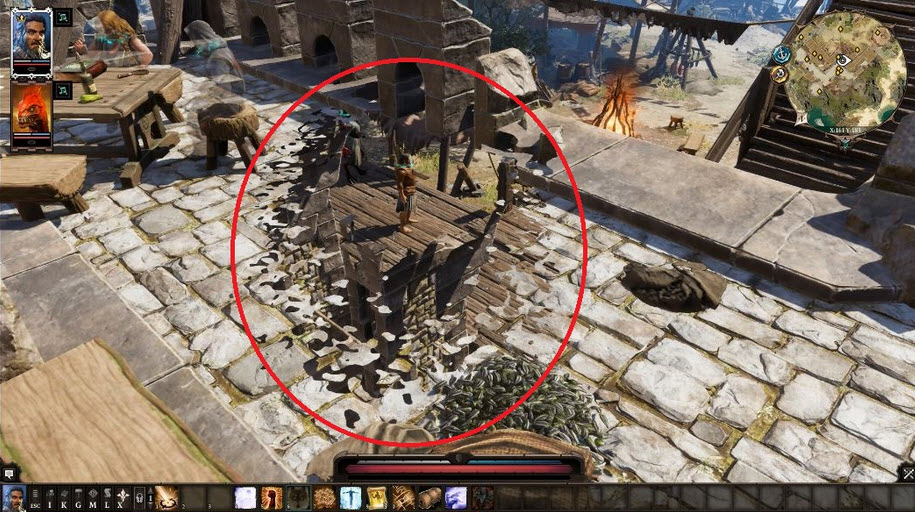To make this effect, you can mask objects by using a stencil Buffer.

the stencil buffer is a general purpose buffer that allows you to store an additional 8bit integer (i.e. a value from 0-255) for each pixel drawn to the screen. Just as shaders calculate RGB values to determine the colour of pixels on the screen, and z values for the depth of those pixels drawn to the depth buffer, they can also write an arbitrary value for each of those pixels to the stencil buffer. Those stencil values can then be queried and compared by subsequent shader passes to determine how pixels should be composited on the screen.

https://docs.unity3d.com/Manual/SL-Stencil.html

https://alastaira.wordpress.com/2014/12/27/using-the-stencil-buffer-in-unity-free/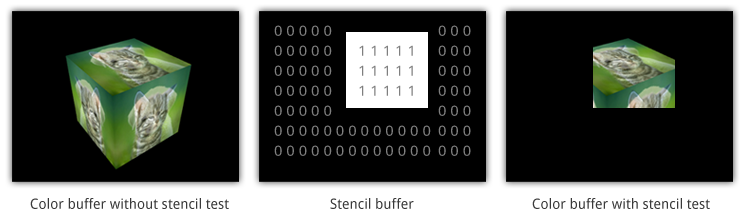Wall Stencil:

Stencil
{
Ref 1 // ReferenceValue = 1
Comp NotEqual // Only render pixels whose reference value differs from the value in the buffer.
}


Stencil
{
Ref 1 // ReferenceValue = 1
Comp Always // Comparison Function - Make the stencil test always pass.
Pass Replace // Write the reference value into the buffer.
}


# Let's Implement.

Shader "Custom/SimpleMask"
{
Properties
{
_MainTex ("Texture", 2D) = "white" {}
_CutOff("CutOff", Range(0,1)) = 0
}
{
LOD 100
Blend One OneMinusSrcAlpha
Tags { "Queue" = "Geometry-1" }  // Write to the stencil buffer before drawing any geometry to the screen
ColorMask 0 // Don't write to any colour channels
ZWrite Off // Don't write to the Depth buffer
// Write the value 1 to the stencil buffer
Stencil
{
Ref 1
Comp Always
Pass Replace
}

Pass
{
CGPROGRAM
#pragma vertex vert
#pragma fragment frag

#include "UnityCG.cginc"

struct appdata
{
float4 vertex : POSITION;
float2 uv : TEXCOORD0;
};

struct v2f
{
float2 uv : TEXCOORD0;
float4 vertex : SV_POSITION;
};

sampler2D _MainTex;
float4 _MainTex_ST;
float _CutOff;

v2f vert (appdata v)
{
v2f o;
o.vertex = UnityObjectToClipPos(v.vertex);

o.uv = TRANSFORM_TEX(v.uv, _MainTex);

return o;
}

fixed4 frag (v2f i) : SV_Target
{
fixed4 col = tex2D(_MainTex, i.uv);
float dissolve = step(col, _CutOff);
clip(_CutOff-dissolve);
return float4(1,1,1,1)*dissolve;
}
ENDCG
}
}
}


use this as the wall:

Shader "Custom/Wall" {
Properties {
_Color ("Color", Color) = (1,1,1,1)
_MainTex ("Albedo (RGB)", 2D) = "white" {}
_Glossiness ("Smoothness", Range(0,1)) = 0.5
_Metallic ("Metallic", Range(0,1)) = 0.0
}
Blend SrcAlpha OneMinusSrcAlpha
Tags { "RenderType"="Opaque" }
LOD 200

Stencil {
Ref 1
Comp NotEqual
}

CGPROGRAM
// Physically based Standard lighting model, and enable shadows on all light types

// Use shader model 3.0 target, to get nicer looking lighting
#pragma target 3.0

sampler2D _MainTex;

struct Input {
float2 uv_MainTex;
};

half _Glossiness;
half _Metallic;
fixed4 _Color;

void surf (Input IN, inout SurfaceOutputStandard o) {
// Albedo comes from a texture tinted by color
fixed4 c = tex2D (_MainTex, IN.uv_MainTex) * _Color;
o.Albedo = c.rgb;
// Metallic and smoothness come from slider variables
o.Metallic = _Metallic;
o.Smoothness = _Glossiness;
o.Alpha = c.a;
}
ENDCG
}
FallBack "Diffuse"
}


# Effect Analysis

If you want to have a procedural texture, you need some noises.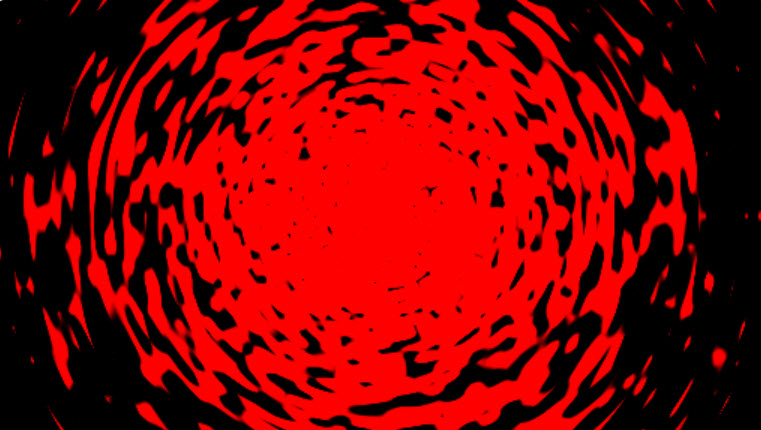you can see this shader in ShaderToy.

To make this effect, instead of using UV Coordinates, use Polar Coordinates then set it to the noise texture.

Uvs are typically layed out in a grid like fashion, like pixels n a screen (X = width, Y = height). Polar coordinates, however, use the x and y a bit differently. One determines how far away from the center of the circle it is and the other determies the degrees, from a 0-1 range, depending on what you need.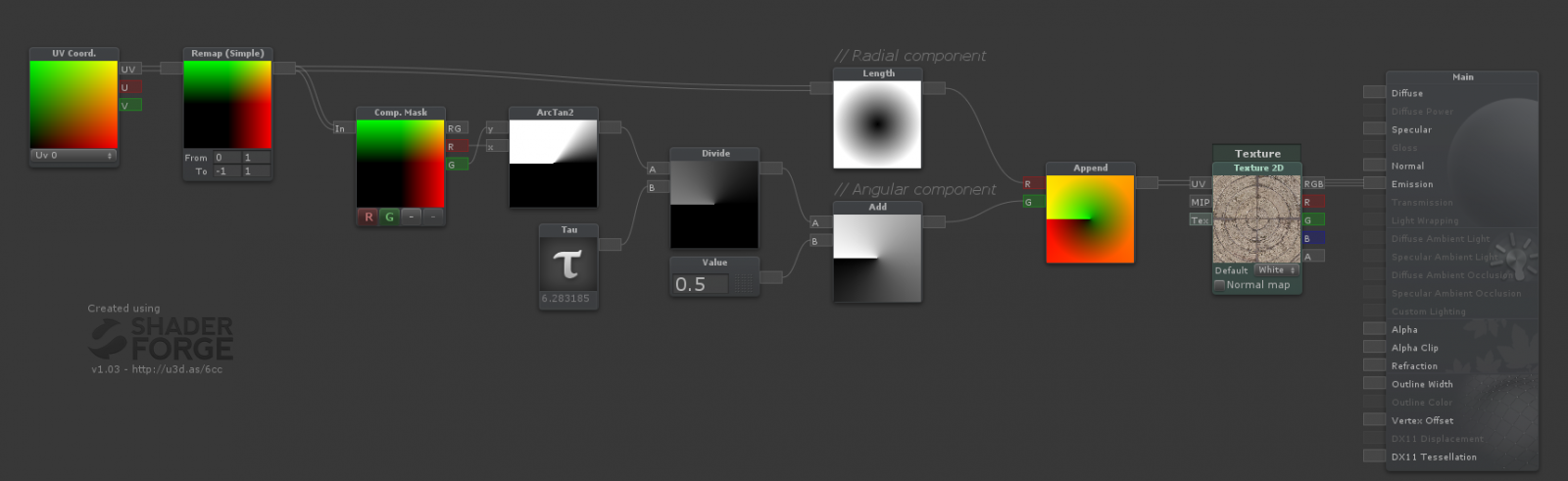Shader "Smkgames/NoisyMask" {
Properties {
_MainTex ("MainTex", 2D) = "white" {}
_Thickness ("Thickness", Range(0, 1)) = 0.25
_Speed("Speed", Float) = 0.5
}
Tags {"Queue"="Transparent" "IgnoreProjector"="true" "RenderType"="Transparent"}
ZWrite Off
Blend SrcAlpha OneMinusSrcAlpha
Cull Off

Pass {
CGPROGRAM
#pragma vertex vert
#pragma fragment frag
#include "UnityCG.cginc"
#pragma target 3.0
uniform sampler2D _MainTex; uniform float4 _MainTex_ST;

struct VertexInput {
float4 vertex : POSITION;
float2 texcoord0 : TEXCOORD0;
};
struct VertexOutput {
float4 pos : SV_POSITION;
float2 uv0 : TEXCOORD0;
float4 posWorld : TEXCOORD1;

};
VertexOutput vert (VertexInput v) {
VertexOutput o = (VertexOutput)0;
o.uv0 = v.texcoord0;

o.pos = UnityObjectToClipPos(v.vertex);
o.posWorld = mul(unity_ObjectToWorld, v.vertex);
return o;
}
float4 frag(VertexOutput i, float facing : VFACE) : COLOR {

float2 uv = (i.uv0*2.0+-1.0); // Remapping uv from [0,1] to [-1,1]
float circleMask = step(length(uv),_NoiseRadius); // Making circle by LENGTH of the vector from the pixel to the center
float circleMiddle = step(length(uv),_CircleRadius); // Making circle by LENGTH of the vector from the pixel to the center
float2 polaruv = float2(length(uv),((atan2(uv.g,uv.r)/6.283185)+0.5)); // Making Polar
polaruv += _Time.y*_Speed/10;
float4 _MainTex_var = tex2D(_MainTex,TRANSFORM_TEX(polaruv, _MainTex)); // BackGround Noise
float3 finalColor = float3(Noise,Noise,Noise);
return fixed4(finalColor+circleMiddle,(finalColor+circleMiddle).r);
}
ENDCG
}
}
FallBack "Diffuse"
}


another solution is using worley noise: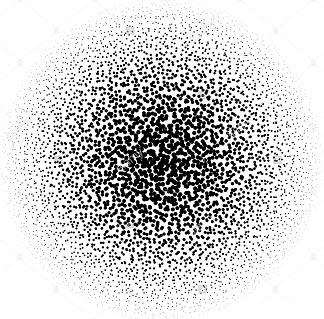# Metaball

then I add the metaball effect from This article: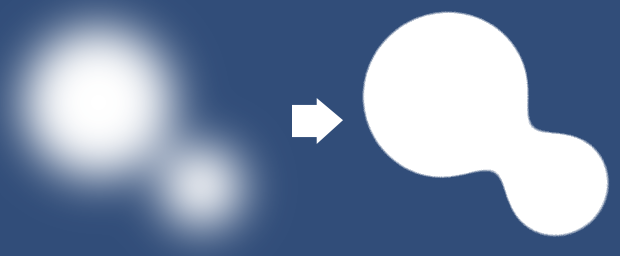# Bill boarding

there is more...

If you want to rotate your mask, to look to your camera, you can use Bill board:

 output.pos = mul(UNITY_MATRIX_P,
mul(UNITY_MATRIX_MV, float4(0.0, 0.0, 0.0, 1.0))
+ float4(input.vertex.x, input.vertex.y, 0.0, 0.0));


this is the mask with Bill boarding:

Shader "Custom/Mask/SimpleMaskBillBoard"
{
Properties
{
_MainTex ("Texture", 2D) = "white" {}
_CutOff("CutOff", Range(0,1)) = 0
_Speed("speed", Float) = 1
_ScaleX ("Scale X", Float) = 1.0
_ScaleY ("Scale Y", Float) = 1.0
}
{
LOD 100
Blend One OneMinusSrcAlpha
Tags { "Queue" = "Geometry-1" }  // Write to the stencil buffer before drawing any geometry to the screen
ColorMask 0 // Don't write to any colour channels
ZWrite Off // Don't write to the Depth buffer

// Write the value 1 to the stencil buffer
Stencil
{
Ref 1
Comp Always
Pass Replace
}

Pass
{
CGPROGRAM
#pragma vertex vert
#pragma fragment frag

#include "UnityCG.cginc"

struct appdata
{
float4 vertex : POSITION;
float2 uv : TEXCOORD0;
};

struct v2f
{
float2 uv : TEXCOORD0;
float4 vertex : SV_POSITION;
};

sampler2D _MainTex;
float4 _MainTex_ST;
float _CutOff;
float _Speed;
float _ScaleX,_ScaleY;

v2f vert (appdata v)
{
v2f o;
o.vertex = mul(UNITY_MATRIX_P,
mul(UNITY_MATRIX_MV, float4(0.0, 0.0, 0.0, 1.0))
+ float4(v.vertex.x, v.vertex.y, 0.0, 0.0)
* float4(_ScaleX, _ScaleY, 1.0, 1.0));

o.uv = TRANSFORM_TEX(v.uv, _MainTex);
return o;
}

fixed4 frag (v2f i) : SV_Target
{
fixed4 col = tex2D(_MainTex, i.uv);
float dissolve = step(col, _CutOff);
clip(_CutOff-dissolve);
return dissolve;
}
ENDCG
}
}
}


# Final result: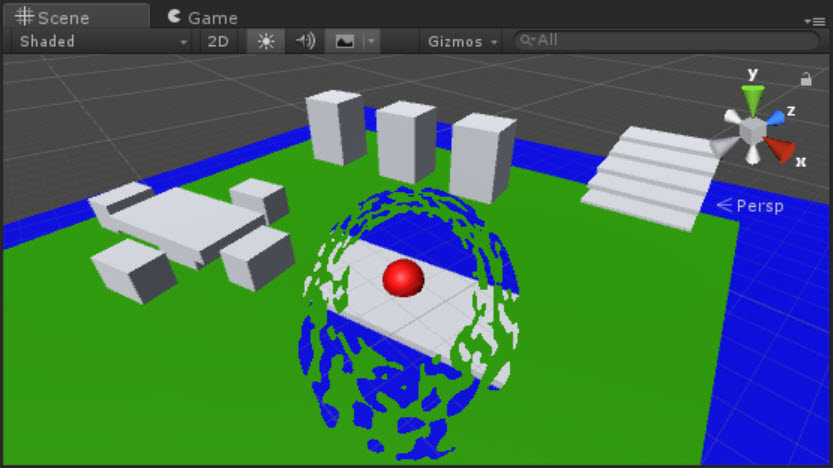source is Available:https://github.com/smkplus/Divinity-Origin-Sin-2

I found a good tutorial that Implemented this effect by Dissolving the world: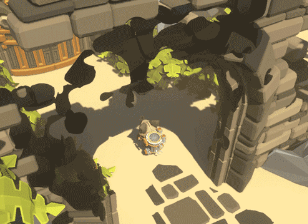Dissolving the world Part 1

Dissolving the world Part 2Shader "Custom/DissolveBasedOnViewDistance" {
Properties{
_MainTex("Albedo (RGB)", 2D) = "white" {}
_Center("Dissolve Center", Vector) = (0,0,0,0)
_Interpolation("Dissolve Interpolation", Range(0,5)) = 0.8
_DissTexture("Dissolve Texture", 2D) = "white" {}
}

Tags { "RenderType" = "Opaque" }
LOD 200

CGPROGRAM

#pragma target 3.0

struct Input {
float2 uv_MainTex;
float2 uv_DissTexture;
float3 worldPos;
float viewDist;
};

sampler2D _MainTex;
sampler2D _DissTexture;
half _Interpolation;
float4 _Center;

// Computes world space view direction
// inline float3 WorldSpaceViewDir( in float4 v )
// {
//     return _WorldSpaceCameraPos.xyz - mul(_Object2World, v).xyz;
// }

void vert(inout appdata_full v,out Input o){
UNITY_INITIALIZE_OUTPUT(Input,o);

half3 viewDirW = WorldSpaceViewDir(v.vertex);
o.viewDist = length(viewDirW);

}

void surf(Input IN, inout SurfaceOutputStandard o) {

float l = length(_Center - IN.worldPos.xyz);

clip(saturate(IN.viewDist - l + (tex2D(_DissTexture, IN.uv_DissTexture) * _Interpolation * saturate(IN.viewDist))) - 0.5);

o.Albedo = tex2D(_MainTex,IN.uv_MainTex);
}
ENDCG
}
Fallback "Diffuse"
}


Another stencil tutorial:Stencil Tutorial

• This is a good explanation of how to do the masking off of the front wall given knowledge of it being in front of the player character, but is there a way to automatically apply this shader only to walls that are in front of the character's geometry? Jan 5, 2018 at 9:17
• @fluffy you can use Raycast for detecting when character is behind of walls then enable Mask. Jan 5, 2018 at 11:38

I'm developing a game called Tales of Tarium which I needed to create a similar effect.Here's the code for the Shader:

Shader "Custom/Dissolve"{
Properties
{
_Color ("Color", Color) = (1,1,1,1)
_MainTex ("Albedo (RGB)", 2D) = "white" {}
_NoiseTex ("Noise (Dissolving)", 2D) = "white" {}
_Metallic("Metallic", 2D) = "black" {}
_NormalMap("Normal", 2D) = "bump" {}
_Occlusion("Occlusion", 2D) = "white" {}
_Emission("Emission ", 2D) = "white" {}
_EmissionIntensity("Emission Intensity", Range(0,10)) = 0.19
_Glossiness ("Smoothness", Range(0,1)) = 0.5
_DissolvePercentage("DissolvePercentage", Range(0,3)) = 0.0
_DistaceMultiplier("DistaceMultiplier", Float) = 1
[Enum(UnityEngine.Rendering.BlendMode)] _SrcBlend ("Src Blend", Float) = 1
[Enum(UnityEngine.Rendering.BlendMode)] _DstBlend ("Dst Blend", Float) = 0
_AlphaCutout("Alpha Cutout", Range(0,1)) = 0.0
}
{
Tags { "RenderType"="Transparent" "Queue"="Transparent"}
LOD 200
CGPROGRAM
#pragma target 3.0

fixed4 _Color;
sampler2D _MainTex;
sampler2D _NoiseTex;
sampler2D _Metallic;
sampler2D _NormalMap;
sampler2D _Occlusion;
sampler2D _Emission;
half _Glossiness;

half _DissolvePercentage;
float _DistaceMultiplier;
float _DistanceToCamera;
float _AlphaCutout;

struct Input
{
float2 uv_MainTex;
float2 uv_NormalMap; INTERNAL_DATA
float3 worldPos;
float3 worldNormal;
float4 screenPos;
};

void surf (Input IN, inout SurfaceOutputStandard o)
{
float pixelDepth = UNITY_Z_0_FAR_FROM_CLIPSPACE(IN.screenPos.z);

float aspectRatio = _ScreenParams.x/_ScreenParams.y;
float2 screenPos = IN.screenPos.xy/IN.screenPos.w;
screenPos.x *= aspectRatio;

half distanceToCenter = 1 - distance(screenPos, float2(0.5*aspectRatio,0.5)) * _DistaceMultiplier;

distanceToCenter = saturate(distanceToCenter);

fixed4 c = tex2D(_MainTex, IN.uv_MainTex);
o.Albedo = c.rgb * _Color;
o.Alpha = 1;
clip(c.a - _AlphaCutout);
fixed4 m = tex2D(_Metallic, IN.uv_MainTex);
o.Metallic = m.rgb;
fixed4 g = tex2D(_Metallic, IN.uv_MainTex);
o.Smoothness = g.rgb * _Glossiness;
o.Occlusion = tex2D(_Occlusion, IN.uv_MainTex);
fixed3 n = UnpackNormal(tex2D(_NormalMap, IN.uv_MainTex));
o.Normal = n;
}
ENDCG
}
FallBack "Diffuse"
}


And you'll need to add this to the player:

using UnityEngine;
public class Player : MonoBehaviour{
private int _id;